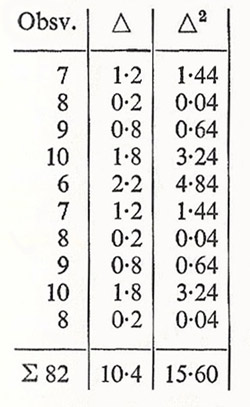A# least squares

The method pf least squares is a technique, developed by Gauss and now widely used in science, for finding the curve which best fits a set of experimental results. It is named after its most important principle; i.e., that for a sequence of measurements the sum of the squares of differences from a particular value is a minimum if that value is taken to be the arithmetic mean of the measurements.

Consider a simple error-calculation made according to the method least squares. Suppose we have a sequence of measurements as shown in the table below.The arithmetic mean of the observations is 8.2, whence we get for the error:

μ = √(15.6/9) = √1.733
m = √(15.6/90) = √0.1733
w = 0.674 √0.1733
δ = 10.4/10 = 1.04 = 0.7979 √0.1733.

Consequently, for the result, we have:

β = 8.2 ± 0.674 √0.1733,

the true value lying inside this range with probability 50%.